﻿ 圆形曲面网板水动力学性能研究

# 圆形曲面网板水动力学性能研究Study on the Hydrodynamic Performance of Circular Cambered Otter Board

Abstract: Trawling is one of the main methods of modern fisheries, of which the single trawling is the common operation types. Otter board is the vital components of a single trawler system; it can provide the desired horizontal opening of a trawl net. In the present study, the hydrodynamic of a circular cambered otter board was studied using flume tank experiment and numerical simulation. The lift/drag coefficient, lift-to-drag ratio and center-of-pressure coefficient were obtained during the data processing, and the flow distribution around the otter board was analyzed according to the numerical results. It showed that the otter board had a good hydrodynamic performance for its high lift coefficient and lift-to-drag ratio, and the camber/deflector structure can delay the flow separation. Numerical simulation results showed a good agreement with experiment ones and could predict the critical angle of attack. Simulation errors the maximum lift coefficient and lift-to-drag ratio were under 10%. Given the otter boards are operated in water, it was suggested to apply both flume tank experiment and numerical simulation to study the hydrodynamic performance of otter board.

1. 引言

2. 材料与方法

2.1. 模型网板

$\frac{{l}_{1}}{{l}_{2}}=s,\text{\hspace{0.17em}}\text{\hspace{0.17em}}\frac{{S}_{1}}{{S}_{2}}={s}^{2},\text{\hspace{0.17em}}\text{\hspace{0.17em}}\frac{{v}_{1}}{{v}_{2}}=\sqrt{s}$ (1)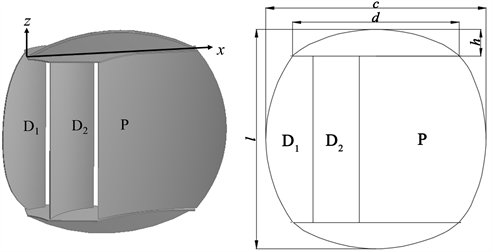Figure 1. Structure of the model otter board (The coordinate frame on it was used to calculate the center-of-pressure of the otter board)Table 1. Detailed parameters of the model otter board

2.2. 试验方法

2.2.1. 水槽试验

2.2.2. 数值模拟Table 2. Parameter settings of the numerical simulation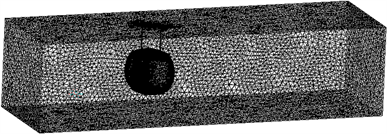Figure 2. Computational grids of the domain during flume tank simulation

2.3. 数据处理

${C}_{L}=\frac{{F}_{L}}{\frac{1}{2}\rho S{v}^{2}}$ (2)

${C}_{D}=\frac{{F}_{D}}{\frac{1}{2}\rho S{v}^{2}}$ (3)

${C}_{mx}=\frac{2{M}_{x}}{\rho S{v}^{2}l}$ (4)

${C}_{mz}=\frac{2{M}_{z}}{\rho S{v}^{2}c}$ (5)

$K=\frac{{C}_{L}}{{C}_{D}}$ (6)

${C}_{pl}=\frac{{d}_{l}}{l}=\frac{{C}_{mx}}{{C}_{L}\mathrm{cos}\alpha +{C}_{D}\mathrm{sin}\alpha }$ (7)

${C}_{pc}=\frac{{d}_{c}}{c}=\frac{{C}_{mz}}{{C}_{L}\mathrm{cos}\alpha +{C}_{D}\mathrm{sin}\alpha }$ (8)

3. 结果

3.1. 网板升力系数

3.2. 网板阻力系数

3.3. 网板升阻比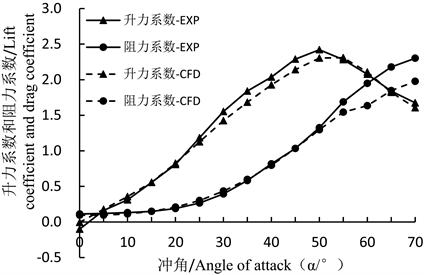Figure 3. The result of flume tank model experiment and numerical simulation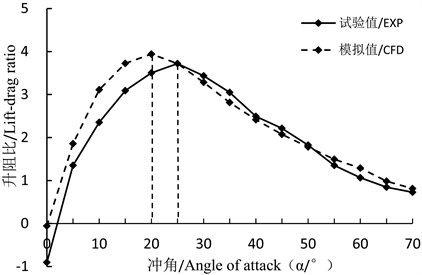Figure 4. Lift-to-drag ratio of the otter board in relation to the angle of attack

3.4. 网板压力中心系数

3.5. 网板周围流场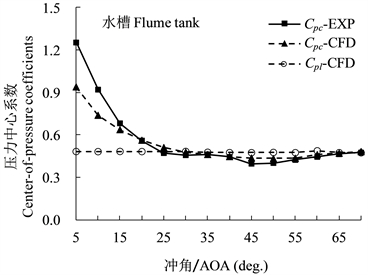Figure 5. Center-of-pressure coefficients of the otter board in relation to the angle of attack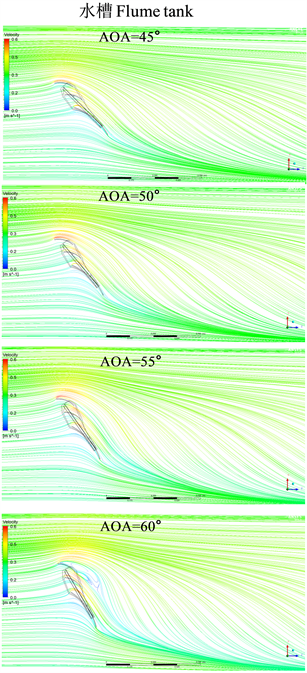Figure 6. Streamlines from a horizontal plane cutting through the center section of the otter board

4. 讨论与结论

4.1. 网板水动力性能

4.2. 数值模拟验证

NOTES

*通讯作者。

 李崇聪, 梁振林, 黄六一, 周为峰, 孙鹏, 王磊. 小型单拖网渔船V型网板水动力性能研究[J]. 海洋科学, 2013(11): 69-73.

 张勋, 王明彦, 徐宝生. 拖网网板型式、结构与性能的研究与应用进展[J]. 中国水产科学, 2004, 11(z1): 107-113.

 虞聪达, 宋伟华. 单船拖网柔性网板的研究(Ⅰ) [J]. 浙江海洋学院学报(自然科学版), 1999, 18(1): 1.

 杨吝. 拖网渔具节能评述[J]. 湛江海洋大学学报, 2000, 20(2): 76-82.

 Sterling D, (2000) The Physical Performance of Prawn Trawling Otter Boards and Low Opening Systems. AME CRC Report, Project 1.4.4, Sterling Trawl Gear Service, Brisbane, 204.

 郭根喜, 刘同渝, 黄小华, 等. 拖网网板动力学理论研究与实践[M]. 广州: 广东科技出版社, 2008: 160-211.

 Broadhurst, M.K., Sterling, D.J. and Cullis, B.R. (2012) Effects of Otter Boards on Catches of an Australian Penaeid Trawl. Fisheries Research, 131-133, 67-75.
https://doi.org/10.1016/j.fishres.2012.07.015

 徐宝生, 张勋, 郁岳峰, 王明彦, 陈裕, 李志国, 等. 矩形V型曲面网板和V型网板生产性对比试验[J]. 海洋渔业, 2006, 28(1): 66-70.

 徐宝生, 张勋, 王明彦. 单船拖网网板的现状及发展趋势[J]. 福建水产, 2010(1): 86-90.

 刘健, 黄洪亮, 陈帅, 吴越, 李灵智, 饶欣. 两种立式曲面V型网板水动力性能的实验研究[J]. 水动力学研究与进展: A辑, 2014, 29(2): 183-188.

 庄鑫, 邢彬彬, 许传才, 张国胜. 网板水动力性能研究综述[J]. 渔业现代化, 2015, 42(5): 63-68.

 刘健, 黄洪亮, 陈帅, 李灵智, 吴越, 徐国栋, 等. 小展弦比立式曲面网板的水动力性能[J]. 水产学报, 2013, 37(11): 1742-1749.

 Kawakami, T. (1953) Mechanical Action of the Otter Board of the Trawl Net. Bulletin of the Japanese Society of Scientific Fisheries, 19, 228-232.
https://doi.org/10.2331/suisan.19.228

 Matuda, K., Hu, F. and Ishizawa, S. (1990) Hydrodynamic Characteristics of Vertical V Type Otter Board. Nippon Suisan Gakkaishi, 56, 1815-1820.
https://doi.org/10.2331/suisan.56.1815

 Wang, L., Wang, L.M., Yu, W., Feng, C., Shi, J., Liu, Y., et al. (2016) Influence of Deflector Angular Variation on Hydrodynamic Performances of Single Slotted Cambered Otter Board. International Forum on Energy, Environment and Sustainable Development, 42, 55-60.
https://dx.doi.org/10.2991/ifeesd-16.2016.95

 Takahashi, Y., Fujimori, Y., Hu, F., Shen, X. and Kimura, N. (2015) Design of Trawl Otter Boards Using Computational Fluid Dynamics. Fisheries Research, 161, 400-407.
https://doi.org/10.1016/j.fishres.2014.08.011

 Xu, Q.C., Huang, L.Y., Zhao, F.F., Wang, X., Tang, Y., Liang, Z., et al. (2017) Study on the Hydrodynamic Characteristics of the Rectangular V-Type Otter Board Using Computational Fluid Dynamics. Fisheries Science, 83, 181-190.
https://doi.org/10.1007/s12562-017-1065-5

 Sala, A., d’Arc Prat Farran, J., Antonijuan, J. and Lucchetti, A. (2009) Performance and Impact on the Seabed of an Existing- and an Experimental-Otter Board: Comparison between Model Testing and Full-Scale Sea Trials. Fisheries Research, 100, 156-166.
https://doi.org/10.1016/j.fishres.2009.07.004

 陈雪忠, 黄锡昌. 渔具模型试验理论与方法[M]. 上海: 上海科学技术出版社, 2011: 388-425.

 夏章英, 卢伙胜, 颜云榕, 等. 应用渔具设计学[M]. 北京: 海洋出版社, 2014: 23-25.

 Xu, Q.C., Huang, L.Y., Zhao, F.F., Wang, X.X., Tang, Y.L., Liang, Z.L., et al. (2017) Effects of Aspect Ratio on the Hydrodynamic Performance of Full-Scale Rectangular Otter Board: Numerical Simulation Study. Ocean Engineering, 142, 338-347.
https://doi.org/10.1016/j.oceaneng.2017.07.007

 Xu, Q.C., Feng, C.L., Huang, L.Y., Xu, J., Wang, L., Zhang, X., et al. (2018) A Comparative Study on Hydrodynamic Performance of Double Deflector Rectangular Cambered Otter Board. Journal of Ocean University of China, 17, 1218- 1224.
https://doi.org/10.1007/s11802-018-3627-2

 Xu, Q.C., Feng, C.L., Huang, L.Y., Xu, J.Q., Wang, L., Zhang, X., et al. (2018) Parameter Optimization of a Double Deflector Rectangular Cambered Otter Board: Numerical Simulation Study. Ocean Engineering, 162, 108-116.
https://doi.org/10.1016/j.oceaneng.2018.05.008

 Xu, Q.C., Huang, L.Y., Liang, Z.L., Zhao, F.-F., Wang, X.-X., Wan, R., et al. (2016) Experimental and Simulative Study on the Hydrodynamics of the Rectangular Otter Board. Proceedings of the 2nd Annual International Conference on Advanced Material Engineering, Wuhan, 15-17 May 2016, 431-437.
https://dx.doi.org/10.2991/ame-16.2016.71

 王明彦, 王锦浩, 张勋, 郁岳峰, 徐宝生. 立式V型曲面网板的水动力性能[J]. 水产学报, 2004, 28(3): 311-315.

Top